International
Tables for
Crystallography
Volume B
Reciprocal space
Edited by U. Shmueli

International Tables for Crystallography (2006). Vol. B, ch. 1.3, p. 64   | 1 | 2 |

Section 1.3.4.2.2.1. Crystallographic groups

G. Bricognea

aMRC Laboratory of Molecular Biology, Hills Road, Cambridge CB2 2QH, England, and LURE, Bâtiment 209D, Université Paris-Sud, 91405 Orsay, France

1.3.4.2.2.1. Crystallographic groups

| top | pdf |

The description of a crystal given so far has dealt only with its invariance under the action of the (discrete Abelian) group of translations by vectors of its period lattice Λ.

Let the crystal now be embedded in Euclidean 3-space, so that it may be acted upon by the group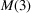of rigid (i.e. distance-preserving) motions of that space. The groupcontains a normal subgroup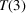of translations, and the quotient group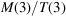may be identified with the 3-dimensional orthogonal group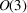. The period lattice Λ of a crystal is a discrete uniform subgroup of.

The possible invariance properties of a crystal under the action ofare captured by the following definition: a crystallographic group is a subgroup Γ ofif

 (i)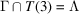, a period lattice and a normal subgroup of Γ; (ii) the factor group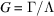is finite.

The two properties are not independent: by a theorem of Bieberbach (1911), they follow from the assumption that Λ is a discrete subgroup ofwhich operates without accumulation point and with a compact fundamental domain (see Auslander, 1965). These two assumptions imply that G acts on Λ through an integral representation, and this observation leads to a complete enumeration of all distinct Γ's. The mathematical theory of these groups is still an active research topic (see, for instance, Farkas, 1981), and has applications to Riemannian geometry (Wolf, 1967).

This classification of crystallographic groups is described elsewhere in these Tables (Wondratschek, 2005), but it will be surveyed briefly in Section 1.3.4.2.2.3for the purpose of establishing further terminology and notation, after recalling basic notions and results concerning groups and group actions in Section 1.3.4.2.2.2.

References

Auslander, L. (1965). An account of the theory of crystallographic groups. Proc. Am. Math. Soc. 16, 1230–1236.
Bieberbach, L. (1911). Über die Bewegungsgruppen der Euklidischen Raume I. Math. Ann. 70, 297–336.
Farkas, D. R. (1981). Crystallographic groups and their mathematics. Rocky Mountain J. Math. 11, 511–551.
Wolf, J. A. (1967). Spaces of constant curvature. New York: McGraw-Hill.
Wondratschek, H. (2005). Introduction to space-group symmetry. In International tables for crystallography, Vol. A. Space-group symmetry, edited by Th. Hahn, Part 8. Heidelberg: Springer.